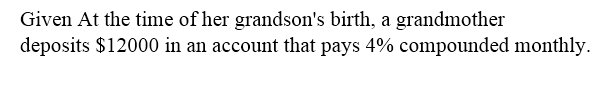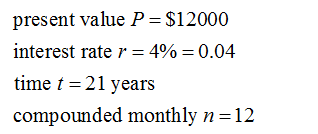# At the time of her​ grandson's birth, a grandmother deposits \$12000 in an account that pays 4% compounded monthly. What will be the value of the account at the​ child's twenty-first​ birthday, assuming that no other deposits or withdrawals are made during this​ period?This is what I have thus far:P=12000r=4% = 0.04t=21 (#of years)n=12 (monthly)A=12000(1+0.04/12)12(21)A=12000*1.003 = 1203612(21)having trouble calculating the exponents. I am getting \$25527.98, but answer is \$27758 and I don't know what I am doing wrong, please help

Question
42 views

At the time of her​ grandson's birth, a grandmother deposits \$12000 in an account that pays 4% compounded monthly. What will be the value of the account at the​ child's twenty-first​ birthday, assuming that no other deposits or withdrawals are made during this​ period?

This is what I have thus far:

P=12000

r=4% = 0.04

t=21 (#of years)

n=12 (monthly)

A=12000(1+0.04/12)12(21)

A=12000*1.003 = 1203612(21)

having trouble calculating the exponents. I am getting \$25527.98, but answer is \$27758 and I don't know what I am doing wrong, please help

check_circle

star
star
star
star
star
1 Rating
Step 1Step 2...

### Want to see the full answer?

See Solution

#### Want to see this answer and more?

Solutions are written by subject experts who are available 24/7. Questions are typically answered within 1 hour.*

See Solution
*Response times may vary by subject and question.
Tagged in

### Algebra